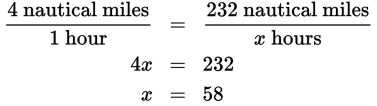# SAT Math Multiple Choice Question 423: Answer and Explanation

### Test Information

Question: 423

3. Water is vital to human health. An average person should consume approximately 2.5 ounces of water per hour. However, because of the salt in it, seawater actually dehydrates the human body and should not be consumed. This is why boats must carry a supply of fresh water when embarking on long trips. Suppose a sailboat is traveling at an average speed of 4 nautical miles per hour with 3 people on board and the trip is 232 nautical miles. What is the minimum number of ounces of water the boat should stock before leaving?

• A. 69.6
• B. 145
• C. 435
• D. 1,113.6

Explanation:

C

Difficulty: Easy

Category: Problem Solving and Data Analysis / Rates, Ratios, Proportions, and Percentages

Strategic Advice: This is a question about rates, so pay careful attention to the units. As you read the question, decide if and when you will need to convert units.

Getting to the Answer: First determine how long it will take the boat to complete the trip. Set up and solve a proportion:The question asks for the total number of ounces of water needed. The recommended rate of consumption is given in ounces per hour, and you now know the number of hours that it will take the boat to complete the trip. Multiply the number of total hours by the number of ounces needed per hour: 58 × 2.5 = 145. Be careful—this isn't the answer. Remember, there are 3 people on board. This is the amount 1 person needs to consume during the trip, so multiply by 3 to get 145 × 3 = 435 ounces.